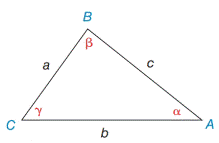Chapter 11.4, Problem 4EElementary Geometry For College St...

7th Edition
Alexander + 2 others
ISBN: 9781337614085

Solutions

Chapter
SectionElementary Geometry For College St...

7th Edition
Alexander + 2 others
ISBN: 9781337614085
Textbook Problem

In Exercises 3 and 4, state the form of the Law of Sines used to solve the problem. Give the answer in a form such as sin   72 ∘ 6.3 = sin   55 ∘ a a) Find β if it is known that b = 8.1 ,   c = 8.4 ,  and  γ = 86 ∘ . b) Find c if it is known that a = 5.3   α = 40 ∘ ,  and  β =80 ∘Exercises 1-8

To determine

a.

To Find:

The angle β by using Law of Sines.

Explanation

Formula:

The Law of Sines,

sinαa=sinβb=sinγc

Where α, β, and γ is the acute angle of the triangle, and a, b, and c is the sides of the triangle.

Calculation:

Given:

In ABC, b=8.1

c=8.4 and

γ=86

To determine

b.

To Find:

The side c by using the Law of Sines.

Still sussing out bartleby?

Check out a sample textbook solution.

See a sample solution

The Solution to Your Study Problems

Bartleby provides explanations to thousands of textbook problems written by our experts, many with advanced degrees!

Get Started

In Exercises 1-6, simplify the expression. 2. 2a23ab9b22ab2+3b3

Applied Calculus for the Managerial, Life, and Social Sciences: A Brief Approach

Describe the scores in a sample that has standard deviation of zero.

Statistics for The Behavioral Sciences (MindTap Course List)

6. Find .

Mathematical Applications for the Management, Life, and Social Sciences

Convert the expressions in Exercises 8596 radical form. 34(1x)5/2

Finite Mathematics and Applied Calculus (MindTap Course List)

Calculate y'. 13. y=e1/xx2

Single Variable Calculus: Early Transcendentals, Volume I

The slope of the tangent line to r = cos θ at is:

Study Guide for Stewart's Single Variable Calculus: Early Transcendentals, 8th